Question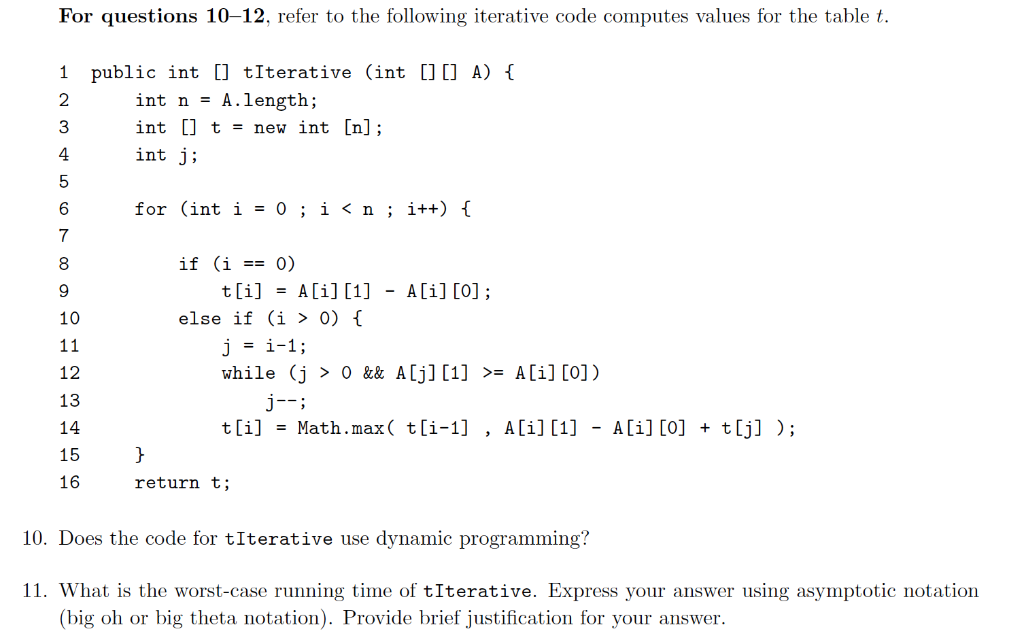10. One major thing to observe in above table that any entry of t say t[ i ] is dependent upon the entry t[i-1] and t[j] where j < i. Since the table t is getting filled in ascending order of indices, hence for calculating t[i], the dependent values t[i-1] and t[j] where j < i are already calculated, hence the above algorithm is based on dynamic programming, where present index value for t is calculated using previous entries.

11. Here outermost for loop run for n iterations while inner "while" loop run for i-1 iterations in worst case.

Hence the statements in innermost loop will run for O(n2) iterations in worst case.

Hence the worst case time complexity will be O(n2).

#### Earn Coins

Coins can be redeemed for fabulous gifts.

Similar Homework Help Questions
• ### (10') 6. For each of the following code blocks, write the best (tightest) big-o time complexity...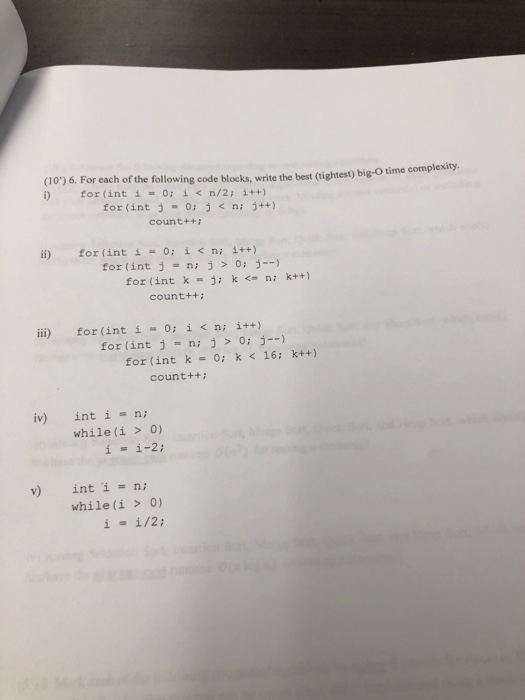(10') 6. For each of the following code blocks, write the best (tightest) big-o time complexity i) for (int i = 0; ǐ < n/2; i++) for (int j -0: ni j++) count++ i) for (int í = 0; i < n; i++) for (int ni j0 - for (int k j k ni kt+) count++ İİİ) for (int í ー 0; i < n; i++) for(int j = n; j > 0; j--) for (int k = 0; k...

• ### Question 3: Given the following two code fragments [2 Marks] (i)Find T(n), the time complexity (as...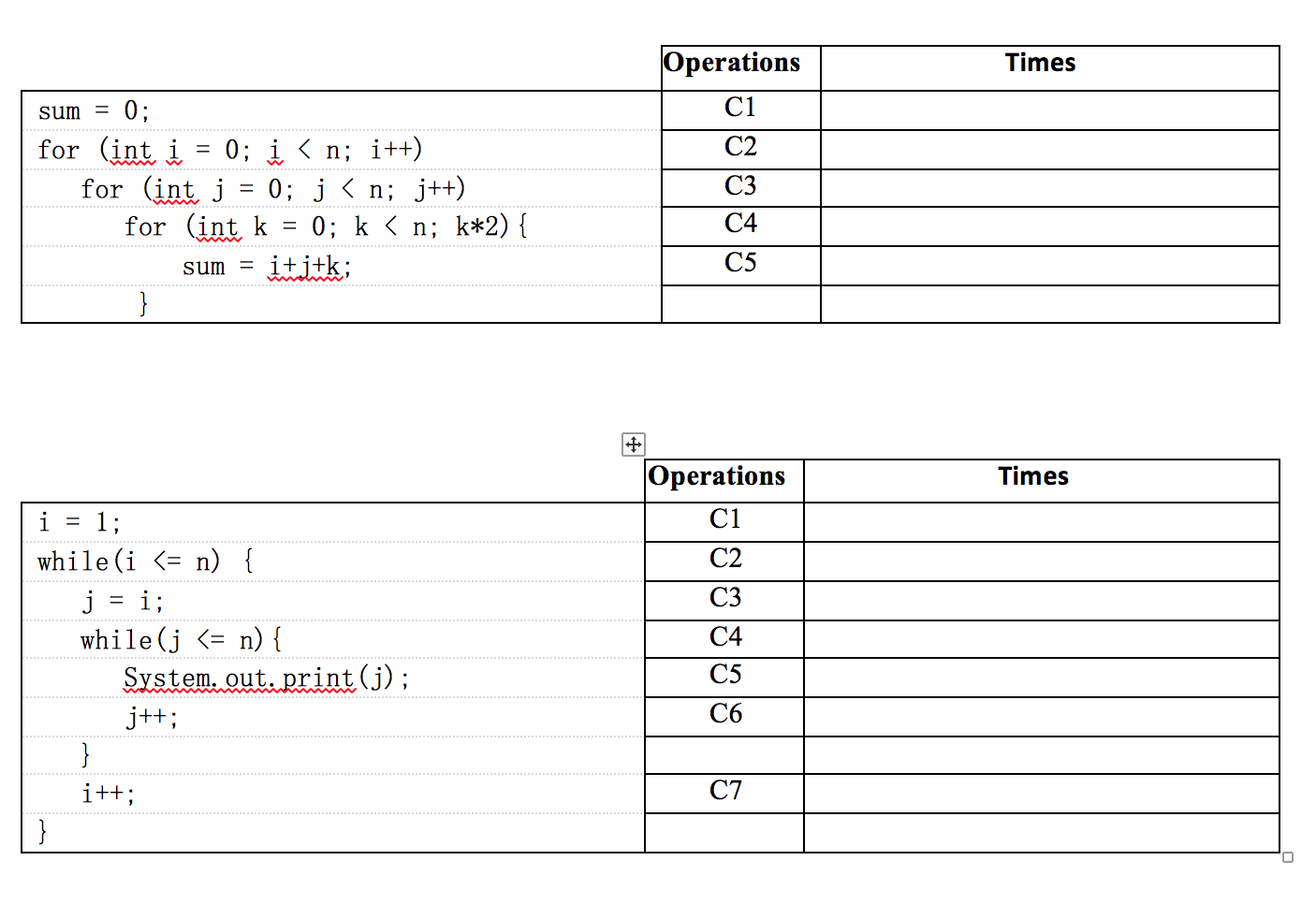Question 3: Given the following two code fragments [2 Marks] (i)Find T(n), the time complexity (as operations count) in the worst case? (ii)Express the growth rate of the function in asymptotic notation in the closest bound possible. (iii)Prove that T(n) is Big O (g(n)) by the definition of Big O (iv)Prove that T(n) is (g(n)) by using limits

• ### II. ALGORITHM COMPLEXITY AND ASYMPTOTIC ANALYSIS The below visual representations of iterative looping structures are provided...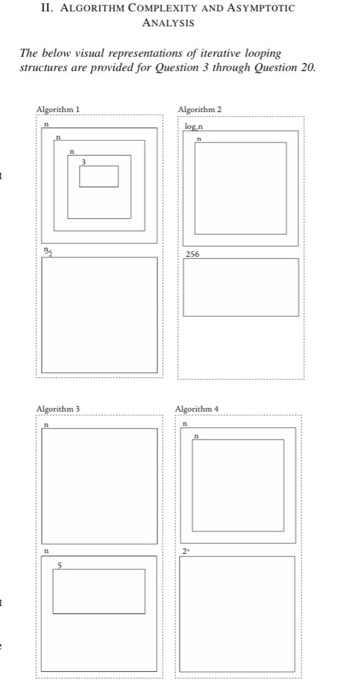II. ALGORITHM COMPLEXITY AND ASYMPTOTIC ANALYSIS The below visual representations of iterative looping structures are provided for Question 3 through Question 20. Algorithm 1 Algorithm 2 log.n 256 Algorithm 3 Algorithm 4 n (10) Match one of our algorithms to the below code snippet. for (int i = 0; i <n; i++) { for(int j = 0; j<n; j++) { for (int k = 0; k<n; k++) { nop++; nop++; nop++; } } } for (int i = 0; i...

• ### What is the Big-Oh order of the following code fragment? The fragment is parametrized on the...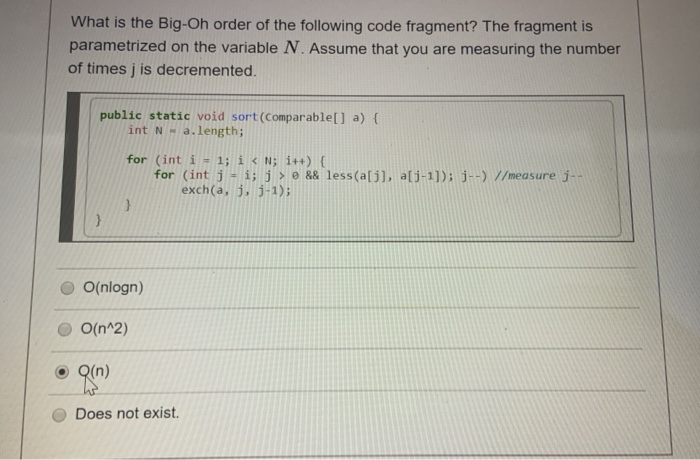What is the Big-Oh order of the following code fragment? The fragment is parametrized on the variable N. Assume that you are measuring the number of times j is decremented. public static void sort(Comparable[] a) { int N-a.length; for (int i = 1; i < N;i++) { for (int j = i; j > && less(a, a[j-1]); j--) //measure j -- exch(a, j, j-1); O(nlogn) O O(n^2) Q(n) Does not exist.

• ### Which big-O expression best characterizes the worst case time complexity of the following code? public static...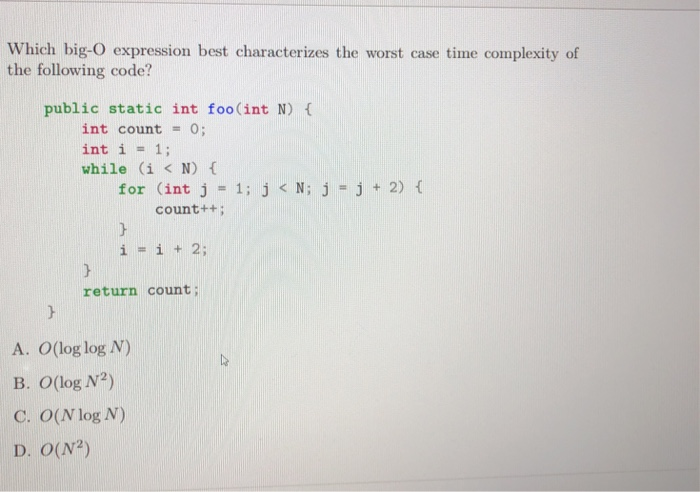Which big-O expression best characterizes the worst case time complexity of the following code? public static int foo(int N) ( int count = 0; int i1; while (i <N) C for (int j = 1; j < N; j=j+2) { count++ i=i+2; return count; A. O(log log N) B. O(log N2) C. O(N log N) D. O(N2)

• ### 1. (25 points) Assume each expression listed below represents the execution time of a program Express...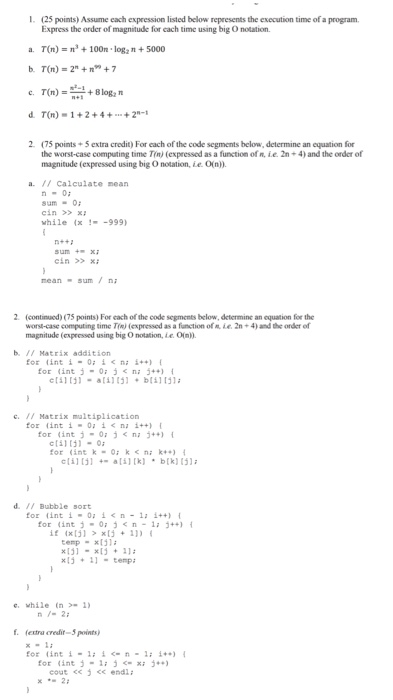1. (25 points) Assume each expression listed below represents the execution time of a program Express the order of magnitude for cach time using big O notation. a. T(n) = ns + 100n . log2 n + 5000 b. T(n) = 2" +n” + 7 d. T(n) 1+2+4+2 2 (75 points+5 extra credit) For cach of the code segments below, determine an equation for the worst-case computing time Tn) (expressed as a function of n, ie. 2n+4) and the order...

• ### find complexity Problem 1 Find out the computational complexity (Big-Oh notation) of the code snippet: Code...

find complexity Problem 1 Find out the computational complexity (Big-Oh notation) of the code snippet: Code 1: for (int i = n; i > 0; i /= 2) { for (int j = 1; j < n; j *= 2) {     for (int k = 0; k < n; k += 2) {               // constant number of operations here     } } } Code 2: Hint: Lecture Note 5, Page 7-8 void f(int n) { if (n...

• ### need help editing or rewriting java code, I have this program running that creates random numbers...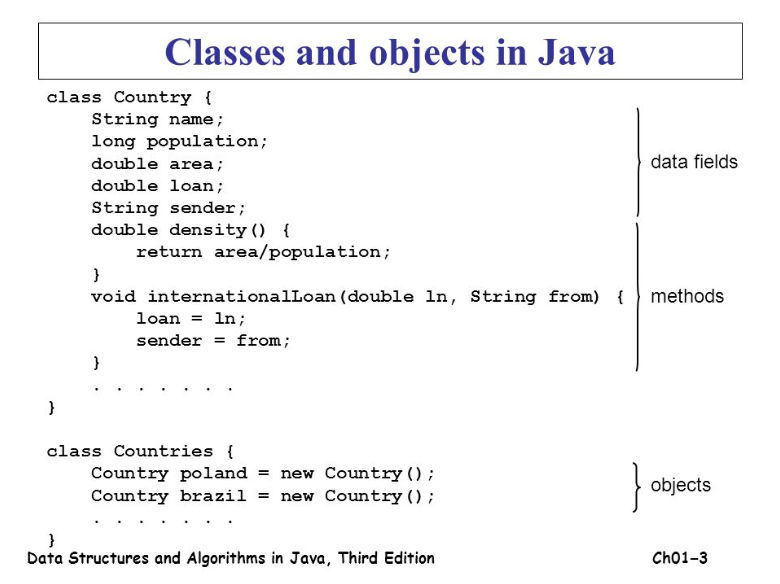need help editing or rewriting java code, I have this program running that creates random numbers and finds min, max, median ect. from a group of numbers,array. I need to use a data class and a constructor to run the code instead of how I have it written right now. this is an example of what i'm being asked for. This is my code: import java.util.Random; import java.util.Scanner; public class RandomArray { // method to find the minimum number in...

• ### Using C++ please explain What is the Big-O time complexity of the following code: for (int...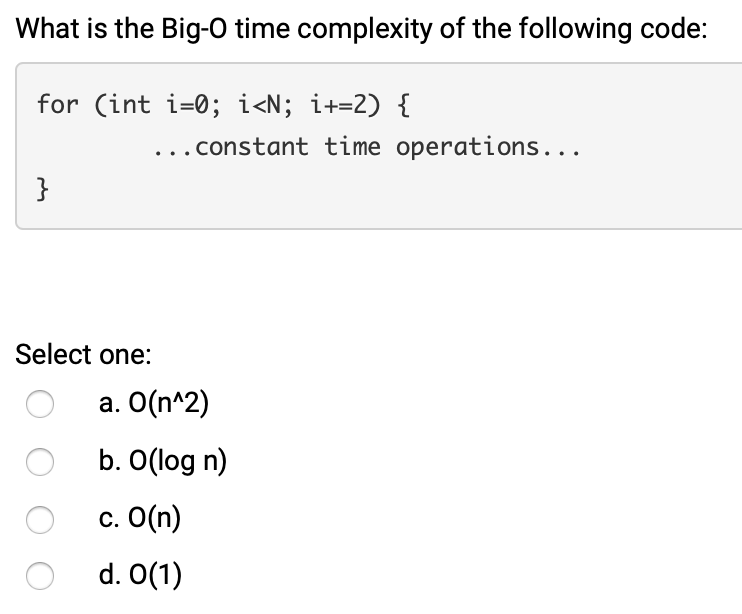Using C++ please explain What is the Big-O time complexity of the following code: for (int i=0; i<N; i+=2) { ... constant time operations... Select one: o a. O(n^2) O b. O(log n) c. O(n) O d. 0(1) What is the Big-O time complexity of the following code: for(int i=1; i<N; i*=2) { ... constant time operations... Select one: O O a. O(n^2) b. 0(1) c. O(n) d. O(log n) O What is the Big-O time complexity of the following...

• ### C++ ONLY! Consider the following code and answer the questions in the table below. #include <iostream>...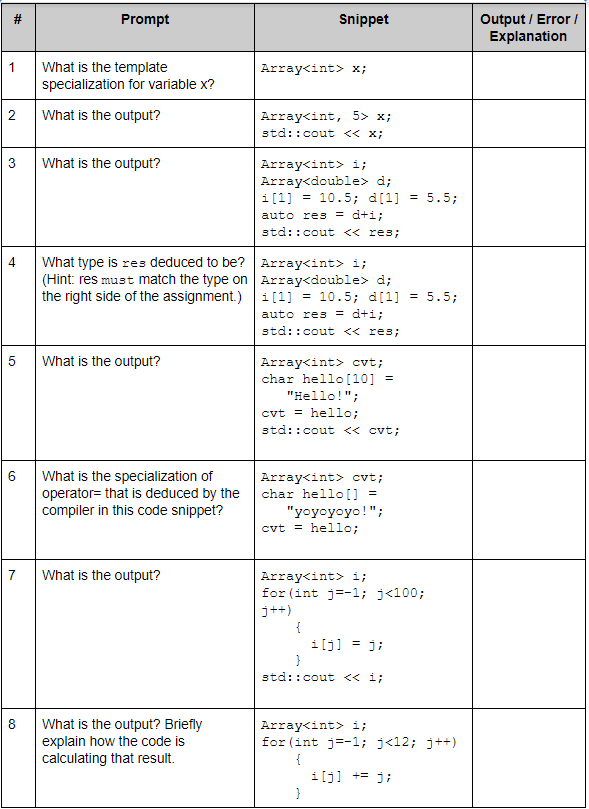C++ ONLY! Consider the following code and answer the questions in the table below. #include <iostream> template <typename T, int N=10> class Array { private:   T array[N+1]; public:   Array() {     for(int i=0; i<N+1; i++) array[i] = 0;   }      T& operator [] (int index) {     if (index>N || index < 0)       return array[N];     else       return array[index];   }   template <typename S>   Array<T,N>& operator= (S &other) {     for(int i=0; i<N+1; i++)       array[i] = other[i];         return *this;   } }; template <typename T, typename...